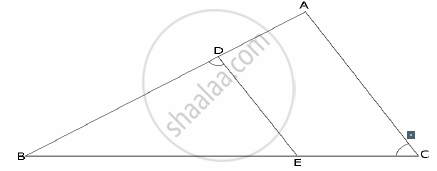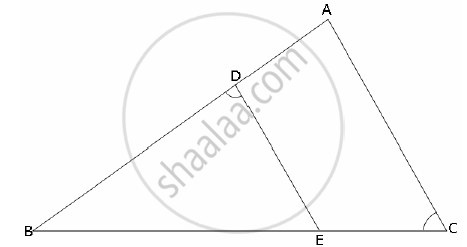# In the Given Figure Abc is a Triangle with ∠Edb = ∠Acb. Prove that δ Abc ~ δ Ebd. If Be = 6 Cm, Ec = 4 Cm, Bd = 5 Cm. and Area of δ Bed = 9 Cm2. Calculate the (I) Length of Ab (Ii) Area of δ Abc - Mathematics

In the given figure ABC is a triangle with ∠EDB = ∠ACB.  Prove that Δ ABC ~ Δ EBD. If BE = 6 cm, EC = 4 cm, BD = 5 cm. And area of Δ BED = 9 cm2. Calculate the

(1) length of AB
(2) area of Δ ABC#### SolutionIn Δ ABC and Δ EBD,
∠ACB = ∠EDB (given)
∠ABC = ∠EBD (common)
∠ABC ~ ∠EBD (by AA- similarity).

1) We have (AB)/(BE) = (BC)/(BD) => AB = (6 xx 10)/5 = 12 cm

2)"Area of Δ ABC"/"Area of Δ BED" = ((AB)/(BE))^2

=> Area of Δ ABC =(12/6)^2 xx 9 cm = 4 xx 9 cm^2 = 36 cm^2

Concept: Similarity of Triangles
Is there an error in this question or solution?